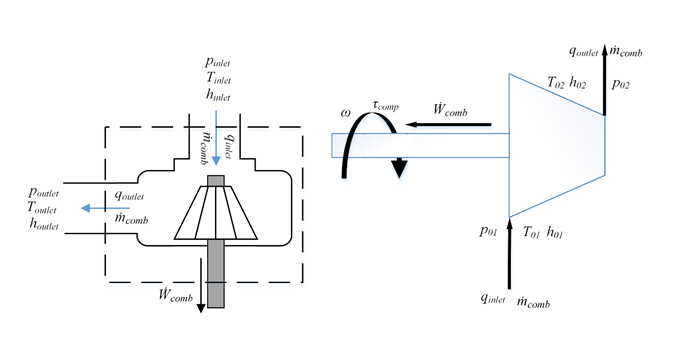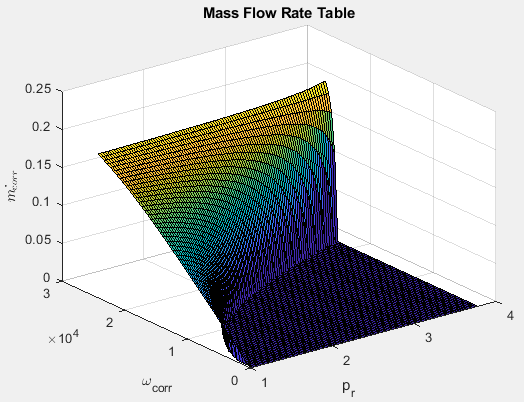# Compressor

Compressor for boosted engines

• Library:
• Powertrain Blockset / Propulsion / Combustion Engine Components / Boost

•## Description

The Compressor block simulates engine boost by using the drive shaft energy to increase the intake manifold pressure. The block is a component of supercharger and turbocharger models. The block uses two-way ports to connect to the inlet and outlet control volumes and the drive shaft. The control volumes provide the pressure, temperature, and specific enthalpy for the compressor to calculate the mass and energy flow rates. To calculate the torque and flow rates, the drive shaft provides the speed to the compressor. Typically, compressor manufacturers provide the mass flow rate and efficiency tables as a function of corrected speed and pressure ratio. You can specify the lookup tables to calculate the mass flow rate and efficiency. The block does not support reverse mass flow.

If you have Model-Based Calibration Toolbox™, click Calibrate Performance Maps to virtually calibrate the mass flow rate and turbine efficiency lookup tables using measured data.

The mass flows from the inlet control volume to the outlet control volume.### Virtual Calibration

If you have Model-Based Calibration Toolbox, click Calibrate Performance Maps to virtually calibrate the mass flow rate and turbine efficiency lookup tables using measured data. The dialog box steps through these tasks.

Description

Import compressor data

Import this compressor data from a file. For more information, see Using Data (Model-Based Calibration Toolbox).

• Mass flow rate, MassFlwRate, in kg/s

• Pressure ratio, PrsRatio, dimensionless

• Efficiency, Eff, dimensionless

The speed, mass flow rate, pressure ratio, and efficiency are in the 2nd-5th columns of the data file, respectively. The first and second rows of the data file provide the variable names and units. For example, use this format.

 Name: Spd MassFlwRate PrsRatio Eff Unit: rad/s kg/s Data: `8373.3` `0.02` `1.21` `0.44` ... ... ... ...

Model-Based Calibration Toolbox limits the speed and pressure ratio breakpoint values to the maximum values in the file.

To filter or edit the data, select . The Model-Based Calibration Toolbox Data Editor opens.

Generate response models

Model-Based Calibration Toolbox fits the imported data to the response models.

Data

Response Model

Mass flow rate

Extended ellipse response model described in Modeling and Control of Engines and Drivelines2

Efficiency

Polynomial

To assess or adjust the response model fit, select . The Model-Based Calibration Toolbox Model Browser opens. For more information, see Model Assessment (Model-Based Calibration Toolbox).

Generate calibration

Model-Based Calibration Toolbox calibrates the response model and generates calibrated tables.

To assess or adjust the calibration, select . The Model-Based Calibration Toolbox CAGE Browser opens. For more information, see Calibration Lookup Tables (Model-Based Calibration Toolbox).

Update block parameters

Update these mass flow rate and efficiency parameters with the calibration.

• Corrected mass flow rate table, mdot_corr_tbl

• Efficiency table, eta_comp_tbl

• Corrected speed breakpoints, w_corr_bpts1

• Pressure ratio breakpoints, Pr_bpts2

### Thermodynamics

The block uses these equations to model the thermodynamics.

CalculationEquations
Forward mass flow

${\stackrel{˙}{m}}_{comp}>0$

${p}_{01}={p}_{inlet}$

${p}_{02}={p}_{outlet}$

${T}_{01}={T}_{inlet}$

${h}_{01}={h}_{inlet}$

First law of thermodynamics

${\stackrel{˙}{W}}_{comp}={\stackrel{˙}{m}}_{comp}{c}_{p}\left({T}_{01}-{T}_{02}\right)$

Isentropic efficiency

${\eta }_{comp}=\frac{{h}_{02s}-{h}_{01}}{{h}_{02}-{h}_{01}}=\frac{{T}_{02s}-{T}_{01}}{{T}_{02}-{T}_{01}}$

Isentropic outlet temperature, assuming ideal gas and constant specific heats

${T}_{02s}={T}_{01}{\left(\frac{{p}_{02}}{{p}_{01}}\right)}^{\frac{\gamma -1}{\gamma }}$

Specific heat ratio

$\gamma =\frac{{c}_{p}}{{c}_{p}-R}$

Outlet temperature

${T}_{02}={T}_{01}+\frac{{T}_{01}}{{\eta }_{comb}}\left\{{\left(\frac{{p}_{02}}{{p}_{01}}\right)}^{\frac{\gamma -1}{\gamma }}-1\right\}$

Heat flows

${q}_{inlet}={\stackrel{˙}{m}}_{comp}{h}_{01}$

${q}_{outlet}={\stackrel{˙}{m}}_{comp}{h}_{02}={\stackrel{˙}{m}}_{comp}{c}_{p}{T}_{02}$

Corrected mass flow rate

${\stackrel{˙}{m}}_{corr}={\stackrel{˙}{m}}_{comp}\frac{\sqrt{{T}_{01}/{T}_{ref}}}{{p}_{01}/{p}_{ref}}$

Corrected speed

${\omega }_{corr}=\frac{\omega }{\sqrt{{T}_{01}/{T}_{ref}}}$

Pressure ratio

${p}_{r}=\frac{{p}_{01}}{{p}_{02}}$

The block uses the internal signal `FlwDir` to track the direction of the flow.

The equations use these variables.

 ${p}_{\text{inlet}}$, ${p}_{01}$ Inlet control volume total pressure ${T}_{inlet}$, ${T}_{01}$ Inlet control volume total temperature ${h}_{inlet}$, ${h}_{01}$ Inlet control volume total specific enthalpy ${p}_{outlet}$, ${p}_{02}$ Outlet control volume total pressure ${T}_{outlet}$ Outlet control volume total temperature ${h}_{outlet}$ Outlet control volume total specific enthalpy ${\stackrel{˙}{W}}_{comp}$ Drive shaft power ${T}_{02}$ Outlet total temperature ${h}_{02}$ Outlet total specific enthalpy ${\stackrel{˙}{m}}_{comp}$ Mass flow rate through compressor ${q}_{inlet}$ Inlet heat flow rate ${q}_{outlet}$ Outlet heat flow rate ${\eta }_{comp}$ Compressor isentropic efficiency ${T}_{02s}$ Isentropic outlet total temperature ${h}_{02s}$ Isentropic outlet total specific enthalpy $R$ Ideal gas constant ${c}_{p}$ Specific heat at constant pressure $\gamma$ Specific heat ratio ${\stackrel{˙}{m}}_{corr}$ Corrected mass flow rate $\omega$ Drive shaft speed ${\omega }_{corr}$ Corrected drive shaft speed ${T}_{ref}$ Lookup table reference temperature ${P}_{ref}$ Lookup table reference pressure ${\tau }_{comp}$ Compressor drive shaft torque ${p}_{r}$ Pressure ratio ${\eta }_{comb,tbl}$ Compressor efficiency 3-D lookup table ${\stackrel{˙}{m}}_{corr,tbl}$ Corrected mass flow rate 3-D lookup table ${\omega }_{corr,bpts1}$ Corrected speed breakpoints ${p}_{r,bpts2}$ Pressure ratio breakpoints

### Power Accounting

For the power accounting, the block implements these equations.

Bus Signal DescriptionEquations

`PwrInfo`

`PwrTrnsfrd` — Power transferred between blocks

• Positive signals indicate flow into block

• Negative signals indicate flow out of block

`PwrDriveshft`

Power transmitted from the shaft

$-{\stackrel{˙}{W}}_{turb}$

`PwrHeatFlwIn`

Heat flow rate at port A

${q}_{outlet}$

`PwrHeatFlwOut`

Heat flow rate at port B

${q}_{outlet}$

`PwrNotTrnsfrd` — Power crossing the block boundary, but not transferred

• Positive signals indicate an input

• Negative signals indicate a loss

`PwrLoss`

Power loss

$-{q}_{inlet}-{q}_{outlet}+{\stackrel{˙}{W}}_{turb}$

`PwrStored` — Stored energy rate of change

• Positive signals indicate an increase

• Negative signals indicate a decrease

Not used

The equations use these variables.

 ${\stackrel{˙}{W}}_{turb}$ Drive shaft power ${q}_{outlet}$ Total outlet heat flow rate ${q}_{inlet}$ Total inlet heat flow rate

## Ports

### Input

expand all

`ShftSpd` — Signal containing the drive shaft angular speed, $\omega$, in rad/s.

Bus containing the inlet control volume:

• `InPrs` — Pressure, ${p}_{\text{inlet}}$, in Pa

• `InTemp` — Temperature, ${T}_{inlet}$, in K

• `InEnth` — Specific enthalpy, ${h}_{inlet}$, in J/kg

Bus containing the outlet control volume:

• `OutPrs` — Pressure, ${p}_{outlet}$, in Pa

• `OutTemp` — Temperature, ${T}_{outlet}$, in K

• `OutEnth` — Specific enthalpy, ${h}_{outlet}$, in J/kg

### Output

expand all

Bus signal containing these block calculations.

SignalDescriptionUnits

`CmprsOutletTemp`

Temperature exiting the compressor

K

`DriveshftPwr`

Drive shaft power

W

`DriveshftTrq`

Drive shaft torque

N·m

`CmprsMassFlw`

Mass flow rate through compressor

kg/s

`PrsRatio`

Pressure ratio

N/A

`DriveshftCorrSpd`

Corrected drive shaft speed

`CmprsEff`

Compressor isentropic efficiency

N/A

`CorrMassFlw`

Corrected mass flow rate

kg/s

`PwrInfo`

`PwrTrnsfrd``PwrDriveshft`

Power transmitted from the shaft

W

`PwrHeatFlwIn`

Heat flow rate at port A

W
`PwrHeatFlwOut`

Heat flow rate at port B

W
`PwrNotTrnsfrd``PwrLoss`

Power loss

W
`PwrStored`

Not used

W

`Trq` — Signal containing the drive shaft torque, ${\tau }_{comp}$, in N·m.

Bus containing:

• `MassFlwRate` — Mass flow rate through inlet,${\stackrel{˙}{m}}_{comp}$, in kg/s

• `HeatFlwRate` — Inlet heat flow rate, ${q}_{inlet}$, in J/s

• `Temp` — Inlet temperature, in K

• `MassFrac` — Inlet mass fractions, dimensionless.

Specifically, a bus with these mass fractions:

• `O2MassFrac` — Oxygen

• `N2MassFrac` — Nitrogen

• `UnbrndFuelMassFrac` — Unburned fuel

• `CO2MassFrac` — Carbon dioxide

• `H2OMassFrac` — Water

• `COMassFrac` — Carbon monoxide

• `NOMassFrac` — Nitric oxide

• `NO2MassFrac` — Nitrogen dioxide

• `NOxMassFrac` — Nitric oxide and nitrogen dioxide

• `PmMassFrac` — Particulate matter

• `AirMassFrac` — Air

• `BrndGasMassFrac` — Burned gas

Bus containing:

• `MassFlwRate` — Outlet mass flow rate, ${\stackrel{˙}{m}}_{comp}$, in kg/s

• `HeatFlwRate` — Outlet heat flow rate, ${q}_{outlet}$, in J/s

• `Temp` — Outlet temperature, in K

• `MassFrac` — Outlet mass fractions, dimensionless.

Specifically, a bus with these mass fractions:

• `O2MassFrac` — Oxygen

• `N2MassFrac` — Nitrogen

• `UnbrndFuelMassFrac` — Unburned fuel

• `CO2MassFrac` — Carbon dioxide

• `H2OMassFrac` — Water

• `COMassFrac` — Carbon monoxide

• `NOMassFrac` — Nitric oxide

• `NO2MassFrac` — Nitrogen dioxide

• `NOxMassFrac` — Nitric oxide and nitrogen dioxide

• `PmMassFrac` — Particulate matter

• `AirMassFrac` — Air

• `BrndGasMassFrac` — Burned gas

## Parameters

expand all

Performance Tables

If you have Model-Based Calibration Toolbox, click Calibrate Performance Maps to virtually calibrate the mass flow rate and turbine efficiency lookup tables using measured data. The dialog box steps through these tasks.

Description

Import compressor data

Import this compressor data from a file. For more information, see Using Data (Model-Based Calibration Toolbox).

• Mass flow rate, MassFlwRate, in kg/s

• Pressure ratio, PrsRatio, dimensionless

• Efficiency, Eff, dimensionless

The speed, mass flow rate, pressure ratio, and efficiency are in the 2nd-5th columns of the data file, respectively. The first and second rows of the data file provide the variable names and units. For example, use this format.

 Name: Spd MassFlwRate PrsRatio Eff Unit: rad/s kg/s Data: `8373.3` `0.02` `1.21` `0.44` ... ... ... ...

Model-Based Calibration Toolbox limits the speed and pressure ratio breakpoint values to the maximum values in the file.

To filter or edit the data, select . The Model-Based Calibration Toolbox Data Editor opens.

Generate response models

Model-Based Calibration Toolbox fits the imported data to the response models.

Data

Response Model

Mass flow rate

Extended ellipse response model described in Modeling and Control of Engines and Drivelines2

Efficiency

Polynomial

To assess or adjust the response model fit, select . The Model-Based Calibration Toolbox Model Browser opens. For more information, see Model Assessment (Model-Based Calibration Toolbox).

Generate calibration

Model-Based Calibration Toolbox calibrates the response model and generates calibrated tables.

To assess or adjust the calibration, select . The Model-Based Calibration Toolbox CAGE Browser opens. For more information, see Calibration Lookup Tables (Model-Based Calibration Toolbox).

Update block parameters

Update these mass flow rate and efficiency parameters with the calibration.

• Corrected mass flow rate table, mdot_corr_tbl

• Efficiency table, eta_comp_tbl

• Corrected speed breakpoints, w_corr_bpts1

• Pressure ratio breakpoints, Pr_bpts2

Corrected mass flow rate lookup table, ${\stackrel{˙}{m}}_{corr,tbl}$, as a function of corrected driveshaft speed, ωcorr, and pressure ratio, pr, in kg/s.Efficiency lookup table, ${\eta }_{comb,tbl}$, as a function of corrected driveshaft speed, ωcorr, and pressure ratio, pr, dimensionless.Corrected drive shaft speed breakpoints, ${\omega }_{corr,bpts1}$, in rad/s.

Pressure ratio breakpoints, ${p}_{r,bpts2}$.

Lookup table reference temperature, ${T}_{ref}$, in K.

Lookup table reference pressure, ${P}_{ref}$, in Pa.

Gas Properties

Ideal gas constant, $R$, in J/(kg*K).

Specific heat at constant pressure, ${c}_{p}$, in J/(kg*K).

 Heywood, John B. Internal Combustion Engine Fundamentals. New York: McGraw-Hill, 1988.

 Eriksson, Lars and Lars Nielsen. Modeling and Control of Engines and Drivelines. Chichester, West Sussex, United Kingdom: John Wiley & Sons Ltd, 2014.